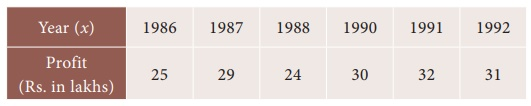Home | | Business Maths 12th Std | Interpolation

# Interpolation

If y is to be estimated for the value of x between two extreme points in a set of values, it is called interpolation.

Interpolation

Consider the profit of a manufacturing company in various years as given belowThe profit for the year 1989 is not available .To estimate the profit for 1989 we use the technique called interpolation. Let x denote the year and y denote the profit. The independent variable x is called the argument and the dependent variable y is called the entry. If y is to be estimated for the value of x between two extreme points in a set of values, it is called interpolation.

## Methods of interpolation

There are two methods for interpolation. One is Graphical method and the other one is algebraic method.

Tags : Numerical Methods , 12th Business Maths and Statistics : Chapter 5 : Numerical Methods
Study Material, Lecturing Notes, Assignment, Reference, Wiki description explanation, brief detail
12th Business Maths and Statistics : Chapter 5 : Numerical Methods : Interpolation | Numerical Methods